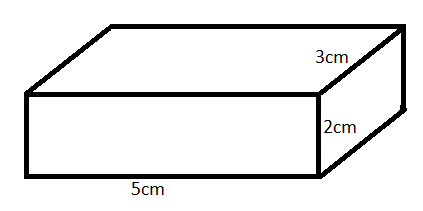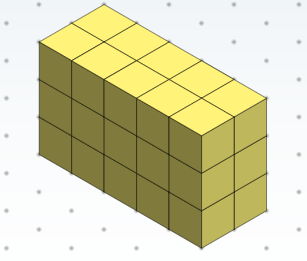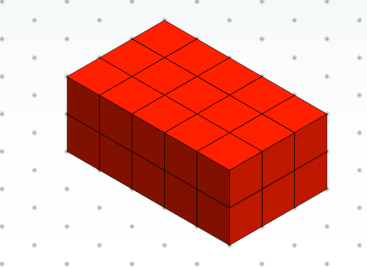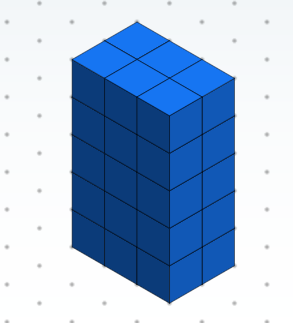QUESTION

# The dimensions of a cuboid are 5 cm, 3 cm and 2 cm. Draw three different isometric sketches of this cuboid.

Hint: For solving this problem, basic knowledge about cuboid and isometric drawing is required. First, we form the cuboid using the dimension given in the question and then and we draw the isometric views.

Complete Step-by-Step solution:
Mathematics is related to all the phenomena occurring in the world. When mathematical structures are good models of real phenomena mathematical reasoning can be used to provide insight or predictions about nature. One of the most important branches of mathematics is geometry. It includes the analysis of figures using simple theorems and results.
In three dimensional figures, one of the most fundamental figures is cuboid. It is composed of length, breadth and height.
One of the common engineering drawing views include isometric view. In this view, a three-dimensional object is shown in two dimensions by using a special paper called isometric paper. In our question, we are given the length, breadth and height of a cuboid as 5 cm, 3 cm and 2 cm. it can be shown as:So, possible three isometric view can be shown as:

(i) $5cm\times2cm\times3cm$(ii) $5cm\times3cm\times2cm$(iii) $3cm\times2cm\times5cm$Note: The key concept involved in solving this problem is the knowledge of cuboid and isometric view. This problem revolves around the concept of isometric projection. It is an important concept for increasing the knowledge of students.